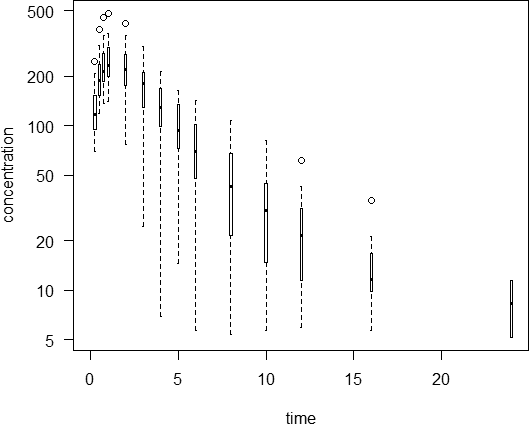## Graphing Mean PK profile [R for BE/BA]

Hi roman_max,

» recently I received a request from Sponsor to represent mean PK profile in a box-and-whiskers fashion with application of a mean connection line in one graph.

The sponsor should re-consider this idea. Box-plots are nonparametric. For log-normal distributed data (which we likely have) the median is an estimate of the geometric mean. If we want to go this way, the arithmetic mean is not a good idea.

» Can anyone share idea (R-code?) how to do it? How a data-set can be organized for this graph?

An idea, yes. I borrowed mittyri’s simulation code. With real data work with the second data.frame.

C <- function(F=1, D, Vd, ka, ke, t) {   C <- F*D/Vd*(ka/(ka - ke))*(exp(-ke*t) - exp(-ka*t))   return(C) } Nsub     <- 24 D        <- 400 ka       <- 1.39 ka.omega <- 0.1 Vd       <- 1 Vd.omega <- 0.2 CL       <- 0.347 CL.omega <- 0.15 t        <- c(seq(0, 1, 0.25), seq(2,6,1), 8,10,12,16,24) ke       <- CL/Vd tmax     <- log((ka/ke)/(ka - ke)) Cmax     <- C(D=D, Vd=Vd, ka=ka, ke=ke, t=tmax) LLOQ.pct <- 2 # LLOQ = 2% of theoretical Cmax LLOQ     <- Cmax*LLOQ.pct/100 df1      <- data.frame(t=t) for (j in 1:Nsub) {   ka.sub <- ka * exp(rnorm(1, sd = sqrt(ka.omega)))   Vd.sub <- Vd * exp(rnorm(1, sd = sqrt(Vd.omega)))   CL.sub <- CL * exp(rnorm(1, sd = sqrt(CL.omega)))   df1    <- cbind(df1, C(D=D, Vd=Vd.sub, ka=ka.sub, ke=CL.sub/Vd.sub, t=t))   df1[which(df1[, j+1] < LLOQ), j+1] <- NA } names(df1)[2:(Nsub+1)] <- paste0("S.", 1:Nsub) df2 <- data.frame(t(df1[-1])) colnames(df2) <- df1[, 1] names(df2) <- t print(signif(df2, 3)) # show what we have plot(x=t, y=rep(0, length(t)), type="n", log="y", xlim=range(t),      ylim=range(df2, na.rm=TRUE), xlab="time",      ylab="concentration", las=1) for (j in seq_along(t)) {   bx <- boxplot(df2[, j], plot=FALSE)   if (bx$n > 0) bxp(bx, log="y", boxwex=0.25, at=t[j], axes=FALSE, add=TRUE) } Which gave in one run:  0 0.25 0.5 0.75 1 2 3 4 5 6 8 10 12 16 24 S.1 NA 199.0 304 353 367 295.0 198.0 128.00 82.2 52.60 21.40 8.75 NA NA NA S.2 NA 132.0 218 269 296 278.0 199.0 129.00 79.7 47.90 16.70 5.65 NA NA NA S.3 NA 82.7 121 136 141 126.0 106.0 88.30 73.5 61.30 42.50 29.50 20.50 9.87 NA S.4 NA 150.0 202 204 184 76.8 24.3 6.91 NA NA NA NA NA NA NA S.5 NA 102.0 167 206 226 216.0 160.0 110.00 72.6 47.30 19.70 8.16 NA NA NA S.6 NA 132.0 213 260 285 285.0 241.0 197.00 160.0 129.00 84.30 55.10 36.00 15.40 NA S.7 NA 96.9 157 193 213 216.0 185.0 152.00 124.0 101.00 67.20 44.50 29.50 13.00 NA S.8 NA 84.1 141 179 203 229.0 213.0 189.00 165.0 143.00 108.00 81.70 61.70 35.20 11.40 S.9 NA 183.0 283 332 349 296.0 211.0 146.00 99.5 67.90 31.50 14.60 6.80 NA NA S.10 NA 93.8 150 181 194 166.0 110.0 66.40 38.6 22.10 7.03 NA NA NA NA S.11 NA 148.0 236 284 304 261.0 176.0 109.00 65.9 39.10 13.60 NA NA NA NA S.12 NA 95.3 156 193 213 206.0 159.0 114.00 79.8 55.30 26.30 12.50 5.89 NA NA S.13 NA 69.3 120 157 182 214.0 197.0 166.00 135.0 108.00 67.40 41.80 25.90 9.91 NA S.14 NA 73.8 118 144 157 157.0 133.0 109.00 89.2 72.70 48.30 32.10 21.30 9.43 NA S.15 NA 245.0 383 453 481 419.0 304.0 213.00 147.0 101.00 48.10 22.90 10.90 NA NA S.16 NA 97.4 157 192 211 204.0 163.0 124.00 93.4 69.90 39.00 21.80 12.20 NA NA S.17 NA 71.9 119 149 167 179.0 160.0 136.00 115.0 96.50 67.90 47.80 33.60 16.60 NA S.18 NA 133.0 203 232 236 159.0 80.0 35.90 15.1 6.10 NA NA NA NA NA S.19 NA 209.0 307 340 338 226.0 127.0 68.00 36.2 19.20 5.39 NA NA NA NA S.20 NA 156.0 235 266 267 172.0 83.5 36.00 14.6 5.66 NA NA NA NA NA S.21 NA 158.0 261 325 360 355.0 274.0 196.00 136.0 92.60 42.50 19.40 8.83 NA NA S.22 NA 97.0 163 206 233 248.0 210.0 165.00 127.0 96.30 54.90 31.20 17.70 5.69 NA S.23 NA 114.0 182 220 240 240.0 208.0 175.00 147.0 123.00 86.70 60.90 42.80 21.20 5.17 S.24 NA 121.0 195 238 261 260.0 216.0 173.00 137.0 109.00 67.90 42.40 26.50 10.40 NA The bad thing is that you have to use very narrow boxes in order to avoid overlaps (boxwex=0.25).In my “bible” that’s called a high ink-to-information ratio* which is bad style. One option would be to draw a thick line instead of the box, a thin line for the whiskers, and smaller points for the outliers, e.g., plot(x=t, y=rep(0, length(t)), type="n", log="y", xlim=range(t), ylim=range(df2, na.rm=TRUE), xlab="time", ylab="concentration", las=1) for (j in seq_along(t)) { bx <- boxplot(df2[, j], plot=FALSE) if (bx$n > 0) {     lines(rep(t[j], 2), c(bx$stats[1, 1], bx$stats[5, 1]))     lines(rep(t[j], 2), c(bx$stats[2, 1], bx$stats[4, 1]), lwd=3, col="gray50")     points(t[j], bx$stats[3, 1], pch=3, cex=0.6) points(rep(t[j], length(bx$out)), bx\$out, pch=1, cex=0.5)   } }Add a line connecting the medians and you are doomed.

• Tufte ER. The Visual Display of Quantitative Information. 2nd ed. Cheshire: Graphics Press; 2001.

Edit: Hey, Shuanghe – you were much faster!

Cheers,
Helmut SchützThe quality of responses received is directly proportional to the quality of the question asked. 🚮
Science QuotesIng. Helmut Schütz